# Row Operations & Reductions with Augmented Matrices

Instructor: Michael Quist

Michael has taught college-level mathematics and sociology; high school math, history, science, and speech/drama; and has a doctorate in education.

If you've ever managed to get hopelessly lost trying to solve a three-variable, three-equation linear system, then this lesson might help a lot. In this lesson, we'll explore how to use matrix row operations to simplify and solve linear systems.

## What is an Augmented Matrix?

Once you've written out the x, y, and z variables for the hundredth time while you're trying to solve linear systems, you begin to realize that it's not about the variables. The only things you're going to work with are the coefficients (products in front of each variable) and the constants (terms with no variable).

Any linear system can be represented as an augmented matrix, which is an array of rows and columns that represent the coefficients and constants present in the original system of equations. Each column in the basic matrix represents one of the variables, and the numbers in the extended column (the one farthest to the right) represent the constants. For example, if a linear system contains the following equations, then the resulting augmented matrix (which we've named 'M') will be as shown.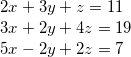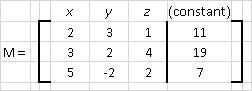Notice that only the coefficients and constant values appear in the matrix (no x's, y's, or z's). Each cell in a matrix has an address: the lowercase version of the matrix name, the row number, and then the column number. Since our matrix is named 'M', for example, then each cell will have a lowercase m followed by the cell's row and column. The top left corner of the matrix would be named m11, and the bottom right cell will be m34.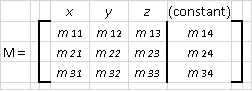## Solving a Linear System of Three Equations Using Matrix Operations

To solve this linear system, we must reduce the rows to the reduced row echelon form, which will look like the figure below but will contain values instead of question marks.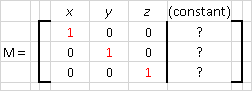Each variable is represented in one of the rows by a 1. This means that the number that appears in the fourth column will be the value for that variable. The value for x will appear in the first row, the value for y in the second row, and the value for z in the third row. Once we find out what numbers should replace the question marks, our work will be done!

To reach the reduced row echelon form we will use two basic operations on the rows in the matrix.

1. We can multiply or divide any row by any value we wish.
2. We can replace any row with a sum of two rows.

In each column, we'll reduce the value for the variable to 1 (first variable, first column and row, second variable, second column and row, etc.), then reduce the other two values in that column to 0's. Okay, here goes!

1. Divide the first row by the value in m11. This will reduce m11 to 1.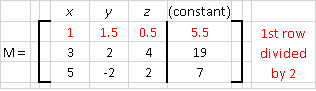2. Reduce cell m21 (first cell in the second row) to 0 by adding two rows together. If we multiply the first row by -3 and then add it to the second row, we'll see m21 go to 0.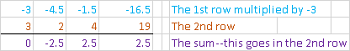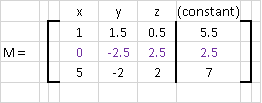3. Reduce cell m31 (first number in the third row) to 0. We'll use the same method we did for m21, this time multiplying the first row by -5, then adding it to the 3rd row.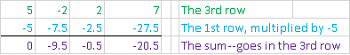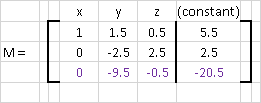4. Set our second 1 in place, this time in the middle column. To do that, we'll divide the second row by -2.5, which is the value in m22. Notice how our second row settles in nicely with all 1's and -1's.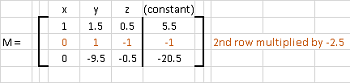5. Now let's set 0's in our first and third rows for the second column. We can clear m12 by multiplying our second row by -1.5 and then adding it to the first row.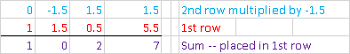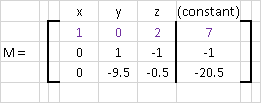6. We'll do the same thing for the third row, using the second row multiplied by 9.5.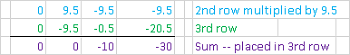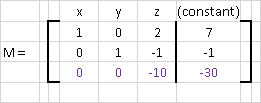We're making progress! One more column to go, and we'll be done.

To unlock this lesson you must be a Study.com Member.

### Register to view this lesson

Are you a student or a teacher?